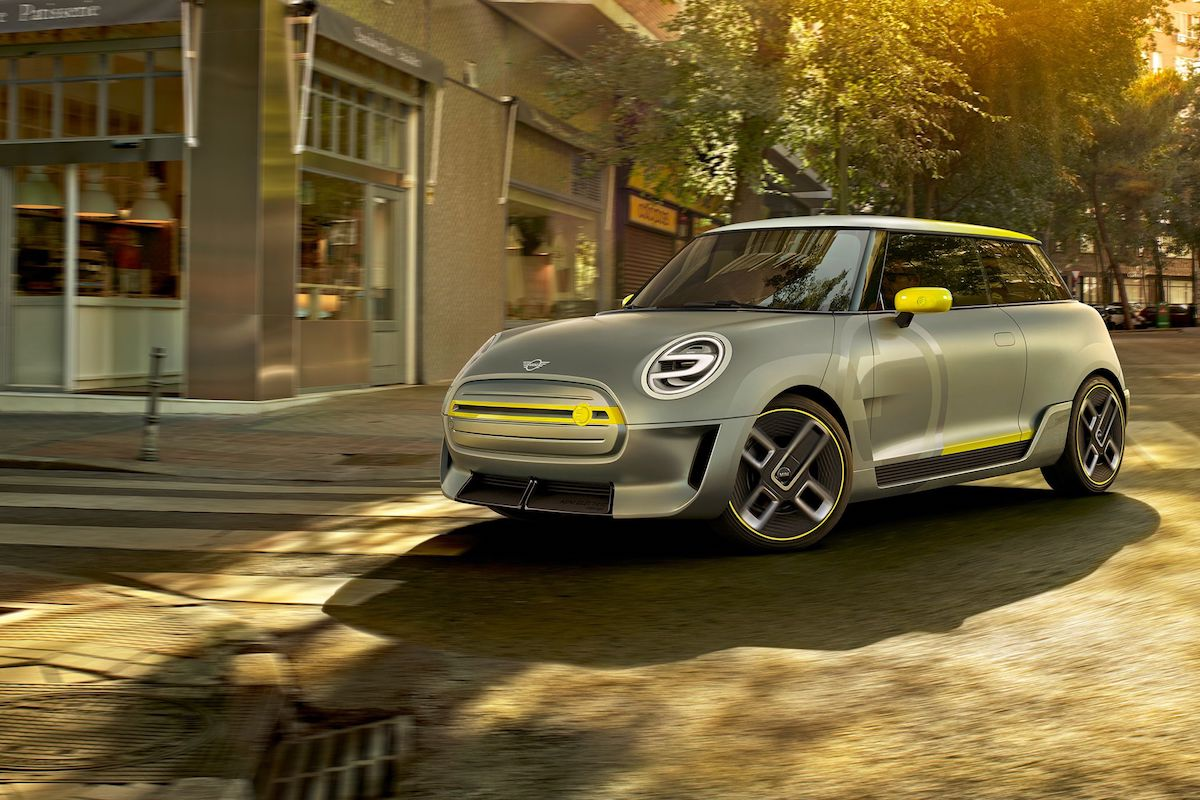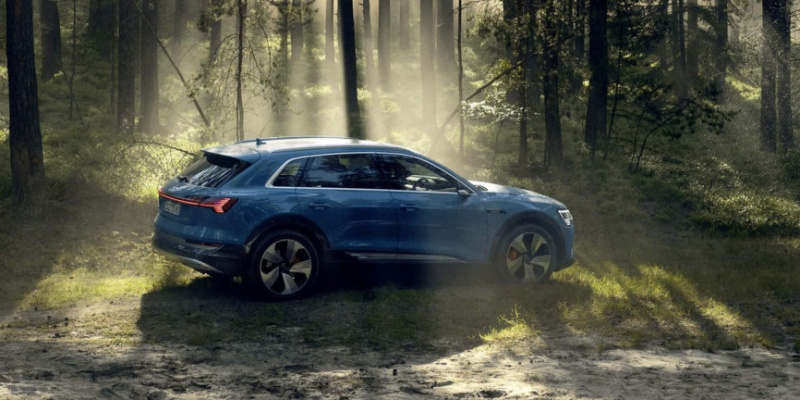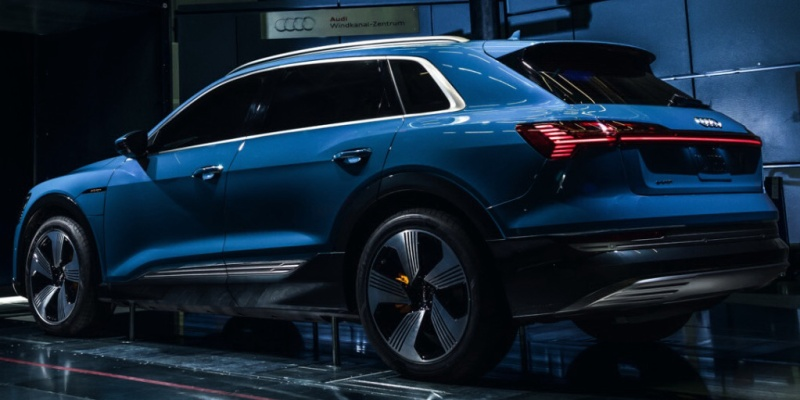Is now the right time to buy an electric car?

Electric cars are always newsworthy, but rarely popular. Does it make sense to buy an electric vehicle right now? Or should you wait for new technology to arrive? Here’s our analysis.• What range do you get from current electric cars? While cheaper models can only offer 100 miles, the latest cars can deliver 300 miles on a full charge.
• What is the cheapest EV? In the U.S., the cheapest EV that looks like an everyday car is the Smart ForTwo Electric Drive, which retails at around \$24,000.
• What are the best electric car accessories? Take a look through our general motoring collection for some useful add-ons.Electric cars are impressive but unpopular

Over time, the benefits of electric car ownership have multiplied. The vehicles are cheaper now, and they offer longer range. In addition, there are many more charging points than there were only five years ago.

Yet for all this progress, the electric car industry seems to be stuck. Only a tiny fraction of new vehicle sales are electric-only, with more drivers opting for hybrid models. Even Tesla is struggling to sustain itself financially.

So, should you buy an electric car now? Or would it be better to wait for the next generation of cars to emerge? Here are the pros and cons.

Why you should buy an electric carIs it worth investing?

Relatively few people own an EV. Even with sales increasing every year, electric vehicles make up less than one percent of all new car sales.

However, the few people who have taken the leap of faith are generally very happy. Tesla has some of the best customer loyalty of any car brand. Similarly, the Nissan Leaf is one of the most popular small cars in terms of owner satisfaction.Owners love the Nissan Leaf

So, why aren’t more electric cars on the road? Demand isn’t the problem. According to a survey by the American Automobile Association, 20 percent of Americans would be tempted to purchase an EV. But the same survey also reveals why people are not buying. Many people hold concerns about reliability, range, and the availability of charging points.Charging is no longer a problem

Mostly speaking, these fears are now misguided. Current models offer impressive range, and there are thousands of fast charging ports across the USA and Europe. While reliability is still a potential weakness, the latest electric cars are fairly solid.

Here are the best electric cars currently available:

Kia e-Niro

Although the eNiro is not quite as handsome as some rivals, this SUV has a massive trunk and a decent range of 282 miles. More impressively, you can fill the batteries to 80 percent full with a fast charger in just 54 minutes.

Price: \$39,667 USD

Hyundai Kona Electric

This practical five-door saloon comes with a 64kWh battery, giving you a range of 300 miles. Fast charging to 80 percent takes 75 minutes, and the Kona Electric is pretty quick off the mark. Just as importantly, the hybrid version of this car has a great safety rating.

Price: \$36,450 USD

Tesla Model S 100D

It goes without saying that Tesla would be on this list. This futuristic saloon has a range of up to 335 miles, and a 17-inch touchscreen console for controlling everything in the car. It also delivers impressive speed and handling, with room for five or seven seats.

Price: \$94,000 USD

Note: these prices do not include Federal or tax rebates, which are available on new electric cars.

Why you should wait

While practical matters certainly weigh heavily on the mind of potential EV buyers, affordability is currently the greatest problem.Expect to pay beween \$30,000 and \$100,000 for a new EV

The running costs of these cars are actually quite reasonable. Electricity is considerable cheaper than liquid fuel, and the difference looks set to increase over time. However, the upfront investment of buying a new electric vehicle is prohibitive for many (even with government incentives).

This may change soon, thanks to extra competition. More than 20 new electric models from top manufacturers will be launching within the next two years. Double that number of hybrids are likely to emerge.

Here are some of the more exciting examples:

Audi E-tron

The E-tron is more than an electric car — it’s a smart car. This SUV has cameras instead of door mirrors, and an electric motor on each axle. It also provides a range of 249 miles, with a top speed of 124 mph. Best of all, it takes only 30 minutes to reach 80 percent battery.

Arriving: Early 2019

Polestar 2

Formerly an offshoot of Volvo, Polestar has something special lined up for 2020. This Scandinavian-made car will have a range of 310 miles, with all-wheel drive. The interior is vegan, and this will be the first car ever to run Android natively. Best of all? It will cost just \$63,000 in the US.

Arriving: July 2020

Mini Electric

At long last, this design icon is about to enter the electric age. Next year, the battery-powered Mini will launch with a range of 200 miles and improved performance. Few other details are known, but it’s already shaping up well.

Arriving: March 2020

Electric car showdown

Do you already own an electric car? Share your experience in the comments!

The Gadget Flow Daily Digest highlights and explores the latest in tech trends to keep you informed. Want it straight to your inbox? Subscribe ➜

Meet Mark Myerson

Mark is best known for writing about apps, but he also loves the tactile, hardware side of technology. Being a professional photographer, he's pretty handy with a camera, and he's a self-confessed tweetaholic.

Click here to tag users that participate in this comment thread.
Click or drag your image here (Maximum Size 4MB, Accepted formats JPG, PNG, GIF).
• 😂
• ❤️
• ♥️
• 😍
• 😭
• 😊
• 😒
• 😘
• 💕
• ☺️
• 😩
• 👌
• 😔
• 😏
• 😁
• 😉
• 👍
• 🙏
• 😌
• 🎶
• 😳
• 🙌
• 🙈
• 😢
• 😎
• ✌️
• 👀
• 😅
• 😴
• 😄
• 💜
• 💔
• 💯
• 😑
• 💖
• 💙
• 😕
• 💁
• 😜
• 😞
• 😋
• 😐
• 😪
• 👏
• 💘
• 💗
• 💞
• ⬅️
• 🙊
• 💋
• 👉
• 🌸
• 😱
• 🔥
• 😡
• 😃
• 🎉
• 👊
• 😫
• 📷
• 🌹
• 😝
• 💪
• 💀
• ☀️
• 💛
• 😤
• 🌚
• 😆
• 😓
• 👈
• ✔️
• 😻
• 😀
• 😷
• 💚
• 👋
• 😣
• 💓
• ▶️
• ◀️
• ↪️
• ↩️
• 👑
• 😚
• 😛
• 😥
• 😇
• 🎧
• 😖
• 😠
• 😬
• 🌟
• 🔫
• 🙋
• 👎
• 💃
• 🎵
• 😶
• 💫
• 👇
• 🔴
• 🙅
• 💥
• 💭
• 👅
• 💩
• 😰
• 💎
• 🙆
• 🍕
• 😹
• 🌞
• 🍃
• 💦
• 🐧
• 💤
• 🚶
• ✈️
• 🎈
• 🎀
• ☑️
• 😟
• 🔞
• 😨
• 🍀
• 🌺
• 🎤
• 👐
• 👻
• 🌴
• ‼️
• 💅
• 👽
• 🙇
• 👼
• 👯
• ❄️
• ☝️
• 😙
• 🌈
• 🌙
• 💟
• 💝
• 🎁
• 🍻
• 😧
• 🌍
• 🎥
• ♣️
• ✖️
• 🏃
• 🌻
• 🌎
• 💐
• 🐶
• 💰
• 🌿
• 👫
• 🍂
• 🌷
• 🎂
• 🐱
• 😵
• 👆
• 😮
• 😯
• 🏀
• 🎄
• 💍
• 🌝
• 😲
• 👭
• 💸
• 😿
• 🙉
• 💨
• 🌵
• ♨️
• ☎️
• 🍁
• 👸
• 💆
• 💌
• 🏆
• 🙍
• 🎊
• 🌼
• 🔪
• 👄
• 🍟
• 🍩
• 😦
• 🌊
• 💣
• 🆗
• 🌀
• 🚀
• 💏
• 🍭
• 🎬
• 🐷
• 😈
• 👿
• 🐝
• 😽
• 💢
• 🎼
• 🎅
• 🌏
• 🏈
• 🎸
• ♦️
• 🐼
• 💬
• 🍓
• 😼
• 🍌
• 🍉
• 😸
• ♠️
• 🔝
• 🍆
• 🔮
• 🍴
• 📲
• 📱
• ⚠️
• 🙀
• 🔸
• 👶
• 🐾
• 👣
• 🍺
• 🍷
• 📹
• 🐰
• 🍹
• 🚬
• 👾
• 🍑
• 🐍
• 🐢
• 🍒
• 😗
• 🐸
• 🌌
• 🚨
• 🐣
• 📕
• 🍬
• 🍔
• 🐻
• 🐯
• 🚗
• 🍦
• 🍍
• 🌾
• 💉
• 🚮
• 🍫
• ▪️
• 📺
• 💊
• 🐙
• 🎃
• 🍇
• 😺
• 💿
• 🍸
• 🍰
• 🎮
• ™️
• ⬇️
• 🚫
• 💄
• 🐳
• 📝
• ®️
• 🍪
• 🐬
• 🔊
• 👨
• 🐥
• 🐒
• 📚
• 👹
• 💂
• 📢
• ✂️
• 👧
• 🎓
• ⚾️
• 🚦
• 👩
• 🎆
• 🌠
• 🆘
• 🍄
• 😾
• 🛅
• 👠
• 🎯
• 🏊
• 🔑
• 👙
• 👪
• 🐘
• 💧
• 🌱
• 🍎
• 🆒
• 📞
• 💵
• 🏡
• 📖
• 💇
• 💻
• 💡
• 🔙
• 👦
• 🔐
• 🙎
• 🍊
• ↔️
• 🌅
• 🍗
• 🔵
• 🚘
• 🍧
• 🐦
• 🌛
• 👓
• 🐐
• 🌃
• 👵
• 🌑
• 👬
• 🛃
• 🐠
• 🏠
• 🔃
• 🌜
• 📍
• 🌕
• 👟
• 🍋
• 🍼
• 🎨
• ✉️
• 🍝
• 🎐
• 🍥
• 🌲
• 🆙
• ⬆️
• ↗️
• ↘️
• ↙️
• 🎭
• 👃
• 🐽
• 🐟
• 👳
• 🐨
• 👂
• ✳️
• 🔹
• 🚿
• 🐛
• 🍜
• 🎩
• 👰
• 🏁
• 🐴
• 🐵
• 🚼
• 🆕
• 🆓
• 🎇
• 🌽
• 🎾
• 🔋
• 🐺
• 🗿
• 🐮
• 📣
• 👴
• 👗
• 🔗
• 🐔
• 🍳
• 🐋
• 🌳
• 🍱
• 📌
• 🔜
• 🔁
• 🐉
• 🐹
• 🏄
• 🐭
• 🌒
• 🚙
• 🅰️
• ⁉️
• 🔌
• 🌓
• 🔱
• 🍞
• 👮
• 🍵
• 🎣
• 🌔
• 🚲
• 👤
• 🍚
• 📻
• 🐤
• ⤵️
• 🌘
• 🌗
• 🔘
• 🐑
• 👱
• 🌖
• 🔒
• 🍏
• 👺
• 🚩
• 🔄
• 🐎
• 🍤
• 🌄
• 🌋
• 🐓
• 📥
• 💒
• 🍣
• 🍨
• 🍅
• 🐇
• ✴️
• 🔺
• 🔆
• 👲
• 🏪
• 👥
• 🐞
• 🔻
• ⤴️
• 📛
• 🛀
• 🐊
• 🌰
• 🐕
• 🐈
• 🔨
• 🍖
• 🐚
• ❇️
• 🅱️
• Ⓜ️
• 🐩
• 🍲
• 👖
• 🍯
• 🎹
• 🔓
• 🗽
• 💲
• 🏂
• 💮
• 👔
• 💠
• 🚺
• 🐜
• 🌇
• 🅾️
• 🐲
• 🐌
• 📀
• 👕
• 🎲
• 🎎
• 🎱
• 🚌
• 🍮
• 🎌
• 〽️
• 🐫
• 🍛
• 🚂
• 🏥
• 🔷
• 🎋
• 🔔
• 🍐
• 🔶
• 🌐
• 🚪
• 🕕
• 🚔
• 📩
• 🌂
• 🎷
• 🚴
• 🍡
• 🏢
• 🚣
• 👒
• 👞
• 🏩
• 🗻
• 🐪
• 👜
• 🎺
• 🏫
• 🐄
• 🌆
• 👷
• 🚽
• 🐖
• 🔰
• 🎻
• 🔛
• 💳
• 🆔
• 🎡
• 🎳
• 💈
• 👛
• 🎢
• 🐀
• 📅
• 🏉
• 🐏
• 🔼
• 🔲
• 📴
• 🗼
• 👘
• 🚢
• 🔎
• 🔍
• 🚒
• 🕦
• 🚓
• 🃏
• 🌉
• 📦
• 🚖
• 📆
• 🏇
• 🐅
• 👢
• 🚑
• 🔳
• 🐗
• 🎒
• 💷
• 🐂
• 🍙
• 🆚
• 🔚
• 🅿️
• 👡
• 💺
• 🚕
• 💼
• 📰
• 🎪
• 🔯
• 🚹
• 🏰
• 🔦
• 🌁
• 🎍
• 🎫
• 🚁
• 💽
• 🚍
• 🍈
• 🏤
• 🔟
• 📓
• 🔕
• 🍢
• 🎏
• 🎠
• 🐡
• 📈
• 🍠
• 🎿
• 🕛
• 📶
• 🚧
• #
• 📡
• 💶
• 👚
• 📒
• 🐆
• 🔅
• 🕒
• 🏬
• 🚚
• 🍶
• 🚃
• 🚤
• 📼
• 🕐
• 🐃
• 🔽
• 💴
• 🔇
• 🎽
• 🕑
• 📎
• 🏧
• 🎦
• 🔭
• 🎑
• 📘
• ◻️
• 📮
• 📧
• 🐁
• 🚄
• 🔩
• 🆖
• 🏨
• 🚾
• 🏮
• 🔂
• 📬
• 📉
• 📗
• 🚜
• 🚇
• 📋
• 📵
• 🕓
• 🚭
• 🎰
• 🕔
• 🛁
• 📜
• 🚉
• 🍘
• 🏦
• 🔧
• 🈯️
• 🚛
• 📄
• 📊
• 🚷
• 📳
• 🕙
• 🕘
• 🚅
• 🚐
• 🚊
• 🕗
• 🈳
• 🚥
• 🚵
• 🔬
• 🏯
• 🔖
• 📑
• 👝
• 🆎
• 📃
• 🎴
• 🕚
• 📠
• 🕖
• 💱
• 🔉
• 💹
• 🆑
• 💾
• 🏣
• 🔈
• 🗾
• 🈺
• 🀄
• 📨
• 📙
• 🚻
• 🈚️
• 🈶
• 📐
• 🚋
• 🈸
• 🚎
• 🈷
• 🔢
• 📔
• 🈲
• 🈵
• 📯
• 🏭
• 🚸
• 🚆
• 📏
• 📟
• 🉑
• 🈴
• 🔏
• 🕜
• 🈂️
• 📤
• 🔀
• 📫
• 🚈
• 🕤
• 🚏
• 📂
• 📁
• 🚰
• 📇
• 🕝
• 🚝
• 🕧
• 🕥
• 🔤
• 📪
• 🕟
• 🚞
• 🚯
• 🕞
• 🕢
• 🕠
• 🔠
• 📭
• 🔣
• 🚡
• 🕣
• 🕡
• 🔡
• 🚠
• 🈁
• 🛂
• 🚱
• 🚟
• 🛄
• 🚳
• 🏳‍🌈
• 🕵
• 🤗
• 🤖
• 🤕
• 🤔
• 🤓
• 🤒
• 🤑
• 🤐
• 🙄
• 🙃
• 🙂
• 🙁
• 🤘
• 🖖
• 🖕
• 🖐
• 🕶
• 👁‍🗨
• 👁
• 🏋
• 🕴
• 🏌
• ❣️
• 🛢
• 🛡
• 🛠
• 🛏
• 🛎
• 🛍
• 🛌
• 🛋
• 🗳
• 🗡
• 🗞
• 🗝
• 🗜
• 🗓
• 🗒
• 🗑
• 🗄
• 🗃
• 🗂
• 🖼
• 🖲
• 🖱
• 🖨
• 🖥
• 🖍
• 🖌
• 🖋
• 🖊
• 🖇
• 🕹
• 🕳
• 🕰
• 🕯
• 📿
• 📽
• 📸
• 🏺
• 🏷
• 🏴
• 🏳
• 🎞
• 🎛
• 🎚
• 🎙
• 🌡
• 🛳
• 🛰
• 🛬
• 🛫
• 🛩
• 🛥
• 🛤
• 🛣
• 🗺
• 🕍
• 🕌
• 🕋
• 🏟
• 🏞
• 🏝
• 🏜
• 🏛
• 🏚
• 🏙
• 🏘
• 🏗
• 🏖
• 🏕
• 🏔
• 🏎
• 🏍
• 🏹
• 🏸
• 🏵
• 🏓
• 🏒
• 🏑
• 🏐
• 🏏
• 🏅
• 🎟
• 🎗
• 🎖
• 🧀
• 🍿
• 🍾
• 🍽
• 🌶
• 🌯
• 🌮
• 🌭
• ☃️
• ☂️
• 🦄
• 🦃
• 🦂
• 🦁
• 🦀
• 🕸
• 🕷
• 🕊
• 🐿
• 🌬
• 🌫
• 🌪
• 🌩
• 🌨
• 🌧
• 🌦
• 🌥
• 🌤
• 🗣
• 🖤
• 🗨
• 🥚
• 🛑
• 🥁